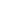### Topics covered in the syllabus are

• Classification of matter
• Scientific method
• Nomenclature and formulas of binary compounds
• Polyatomic ions and other compounds
• Determination of atomic masses
• Mole concept
• Percent composition
• Empirical and molecular formula
• Writing chemical equations and drawn representations
• Balancing chemical equations
• Applying mole concept to chemical equations (stoichiometry)
• Determining limiting reactants, theoretical and percent yield of reactions
• Electrolytes and properties of water
• Molarity and preparation of solutions
• Redox and single replacement reactions
• Double replacement reactions
• Combustion reactions
• Decomposition reactions
• Precipitation reactions and solubility rules
• Acid-Base reactions and formation of a salt by titration
• Balancing redox reactions
• Simple redox titrations
• Electron configuration and the Aufbau principle
• Valence electrons and Lewis dot structures
• Periodic trends
• Table arrangement based on electronic properties
• Properties of light and study of waves
• Atomic spectra of hydrogen and energy levels
• Quantum mechanical model
• Quantum theory and electron orbitals
• Orbital shape and energies
• Spectroscopy
• Lewis Dot structures
• Resonance structures and formal charge
• Bond polarity and dipole moments
• VSEPR models and molecular shape
• Polarity of molecules
• Lattice energies
• Hybridization
• Molecular orbitals and diagrams
• Electron configuration and the Aufbau principle
• Valence electrons and Lewis dot structures
• Periodic trends
• Table arrangement based on electronic properties
• Properties of light and study of waves
• Atomic spectra of hydrogen and energy levels
• Quantum mechanical model
• Quantum theory and electron orbitals
• Orbital shape and energies
• Spectroscopy
• Law of conservation of energy, work, and internal energy
• Endothermic and exothermic reactions
• Potential energy diagrams
• Calorimetry, heat capacity, and specific heat
• Hess's Law
• Heat of formation/combustion
• Bond energies
• Measurement of gases
• General gas laws - Boyle, Charles, Combined, and Ideal
• Dalton's Law of partial pressure
• Molar volume of gases and stoichiometry
• Graham's Law
• Kinetic Molecular Theory
• Real gases and deviation from ideal gas law
• Graham's law demonstration
• Law of conservation of energy, work, and internal energy
• Endothermic and exothermic reactions
• Potential energy diagrams
• Calorimetry, heat capacity, and specific heat
• Hess's Law
• Heat of formation/combustion
• Bond energies
• Structure and bonding
• Metals, network, and molecular
• Ionic, hydrogen, London, van der Waals
• Vapor pressure and changes in state
• Heating and cooling curves
• Composition of solutions
• Colloids and suspensions
• Separation techniques
• Effect on biological systems
• Rates of reactions
• Factors that affect rates of reactions/ collision theory
• Reaction Pathways
• Rate equation determination
• Rate constants
• Mechanisms
• Method of initial rates
• Integrated rate laws
• Activation energy and Boltzmann distribution
• Characteristics and conditions of chemical equilibrium
• Equilibrium expression derived from rates
• Factors that affect equilibrium
• Le Chatelier's principle
• The equilibrium constant
• Solving equilibrium problems
• Definition and nature of acids and bases
• Kw and the pH scale
• pH of strong and weak acids and bases
• Polyprotic acids
• pH of salts
• Structure of Acids and Bases
• Characteristics and capacity of buffers
• Titrations and pH curves
• Choosing Acid-Base Indicators
• pH and solubility
• Ksp Calculations and Solubility Product
• Laws of thermodynamics
• Spontaneous process and entropy
• Spontaneity, enthalpy, and free energy
• Free energy
• Free energy and equilibrium
• Rate and Spontaneity
• Balancing redox equations
• Electrochemical cells and voltage
• The Nernst equation
• Spontaneous and non-spontaneous equations
• Chemical applications